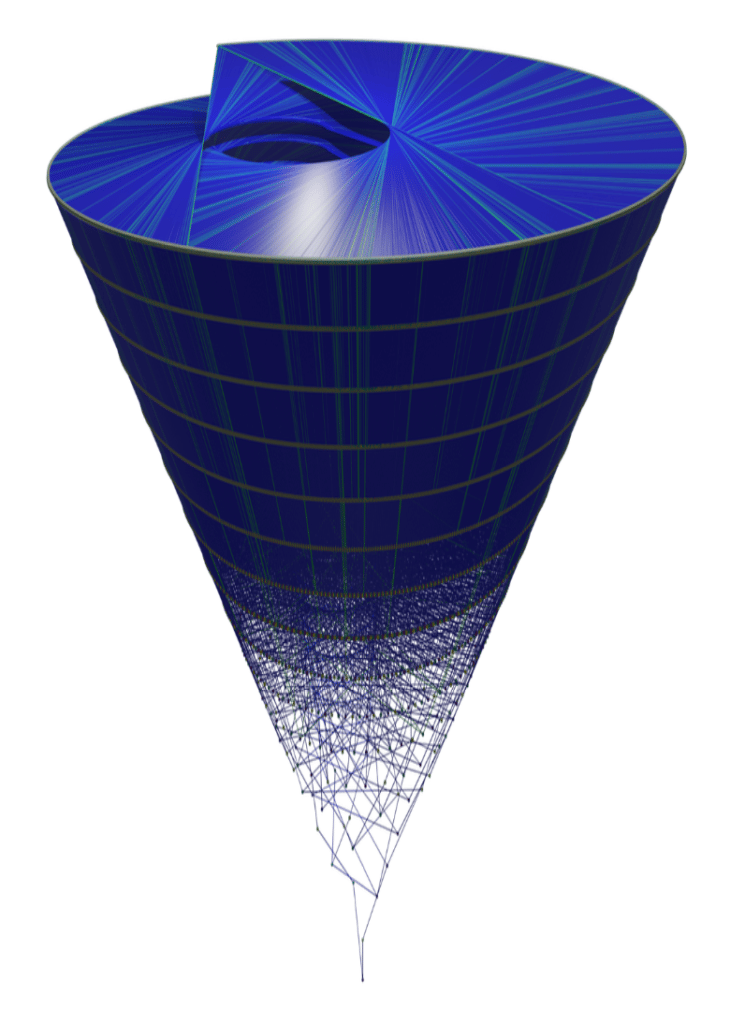# “An Algorithm for Linearizing the Collatz Convergence”Abstract

The Collatz dynamic is known to generate a complex quiver of sequences over natural numbers for which the inflation propensity remains so unpredictable it could be used to generate reliable proof-of-work algorithms for the cryptocurrency industry; it has so far resisted every attempt at linearizing its behavior. Here, we establish an ad hoc equivalent of modular arithmetics for Collatz sequences based on five arithmetic rules that we prove apply to the entire Collatz dynamical system and for which the iterations exactly define the full basin of attractions leading to any odd number. We further simulate these rules to gain insight into their quiver geometry and computational properties and observe that they linearize the proof of convergence of the full rows of the binary tree over odd numbers in their natural order, a result which, along with the full description of the basin of any odd number, has never been achieved before. We then provide two theoretical programs to explain why the five rules linearize Collatz convergence, one specifically dependent upon the Axiom of Choice and one on Peano arithmetic.